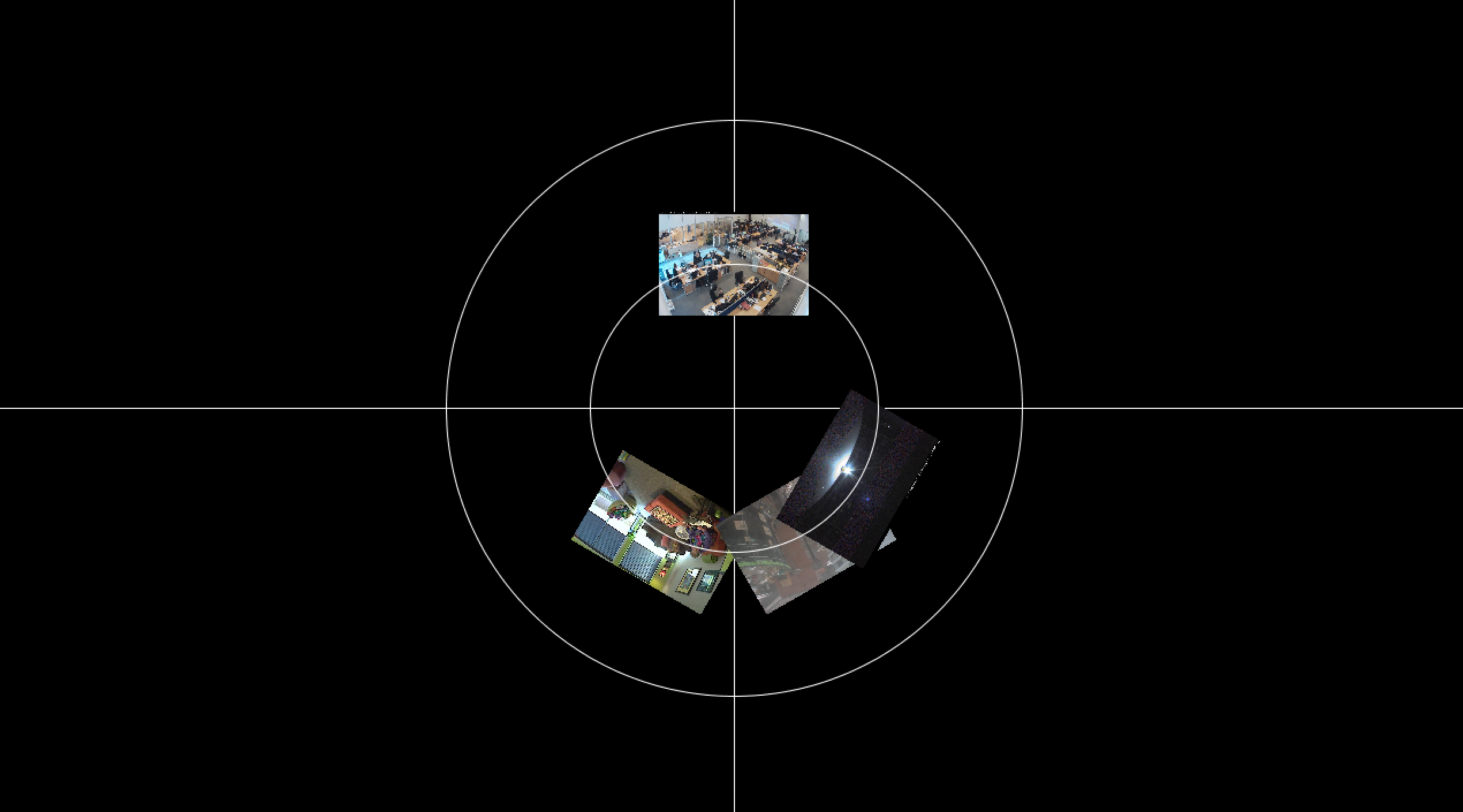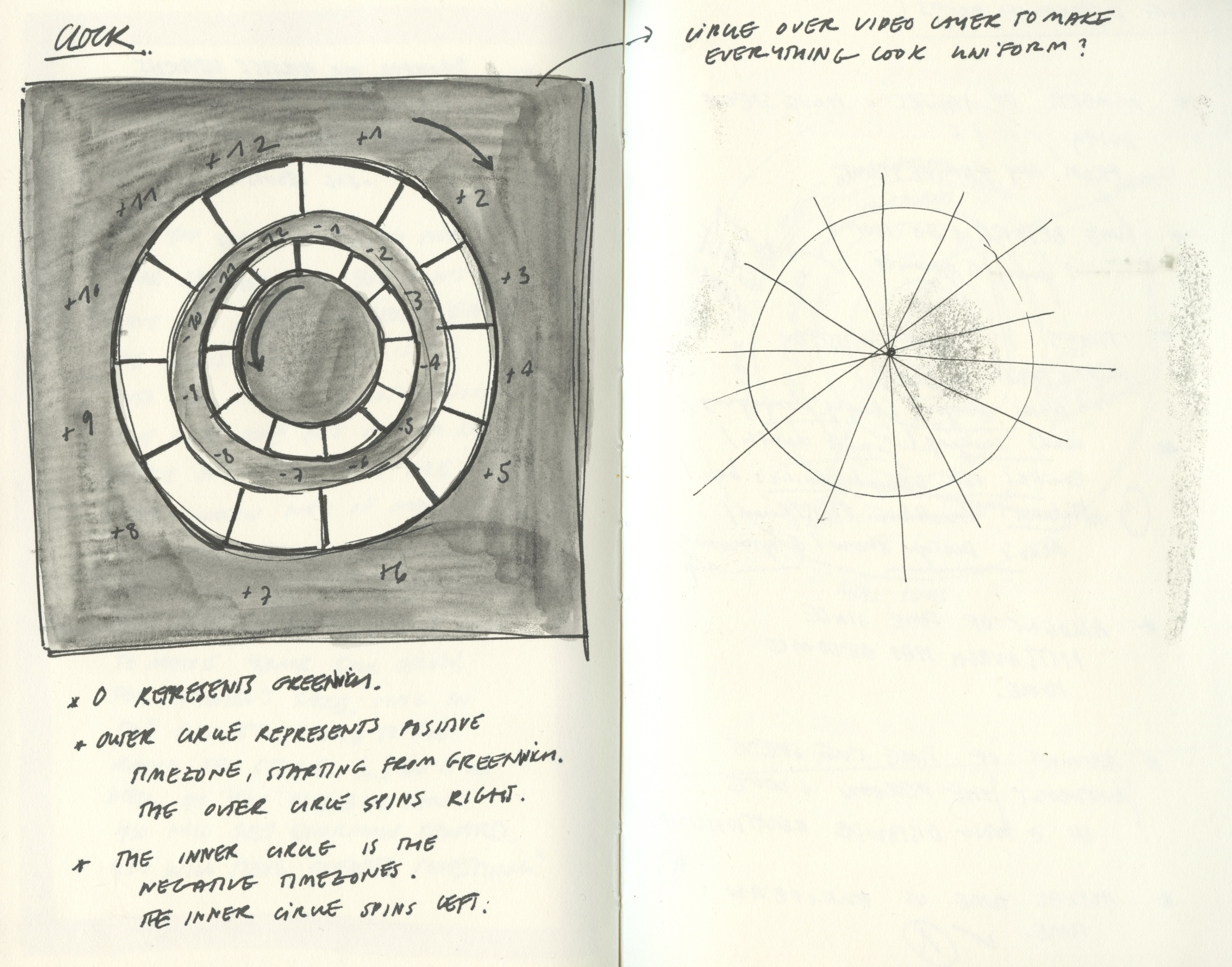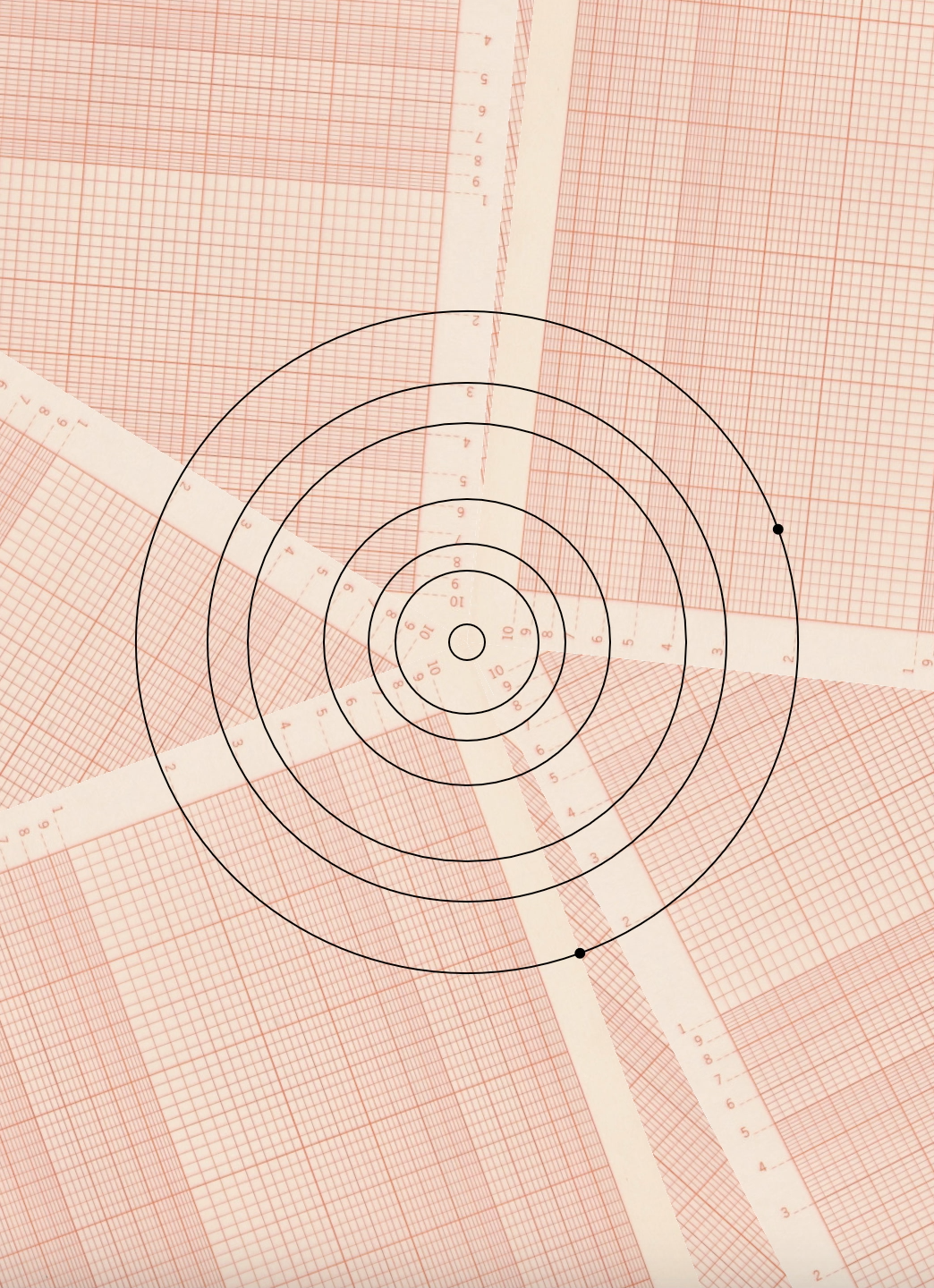# dechoes – ClockGif of the clock. The code could not be correctly embedded due to an unauthorized image loading of some sort.

My goal for this clock was to try and represent time distance instead of actual time. I have spent my entire life far in time (and space) from people I care for, but more so than physical distance, it is the emotional one that bothers me. Time difference makes communication and emotional involvement extremely draining. This piece is a data visualization of different personal emotional events that have been affected by time difference:

Circles, from outer-most to inner-most:

1. US time vs. EU time (hours and minutes).
2. Days elapsed since I’ve been home last.
3. Number of big life events I have missed since being 6h away in time. This includes surgeries, graduations, emotional growth and death.
4. Countdown of the number of days that Pittsburgh has felt like home, represented in seconds instead of days.
5. Countdown of the number of days I spent in a long distance relationship, represented in seconds instead of days.
6. Seconds counter.

Here is the functioningclock with a simple orange background, to show its effect in real time:

I am pretty satisfied with how this turned out, however I feel like I could have done something so much more interesting if I had more time. This topic is one that matters to me on a personal level, and I would have liked to have done it justice. I struggled a little in getting the countdown timer to work, but I think I was able to learn a lot because of it.

Preliminary sketches:Process Pictures:

The first two pictures are sketches and first iteration I had made for a another clock idea, which involved scrapping live webcams from different time zones.Code:

```//Huge thanks to Kabir for helping me debug, //completely unsolicited, during his lunch break //And as per usual, thanks to Golan for helping me, //despite my fucked-up keyboard // Must make a local server // in terminal: python -m SimpleHTTPServer // in browser: http://localhost:8000/Desktop/Reminders/   var img; var canvasX = 530; var canvasY = 728;   //var madridArray = [];   // Angle and angular velocity, accleration var theta = 0; var theta2 = 0; var theta3 = 0; var theta4 = 0; var theta5 = 0; var theta6 = 0; var r = 0; var r2 = 0; var r3 = 0; var r4 = 0; var r5 = 0; var r6 = 0; var startTime = 0; var timeElapsed = 0;     //Sets the spot where we want our point to appear var thetaOffset = 0; var thetaOffset2 = 0;     var now = new Date(); var start = new Date(now.getFullYear(), 0, 0); var diff = now - start; var oneDay = 1000 * 60 * 60 * 24; var dayOfYear = Math.floor(diff / oneDay);   var begin; var duration = 138; var time = 138; var duration2 = (547 + dayOfYear); var time2 = (547 + dayOfYear); var duration3 = 9; var time3 = 9;       //-----------------------------------------------------------   function preload() { img = loadImage('data/graphPaper.png'); }     function setup() { createCanvas(canvasX, canvasY);   // ROTATION: the radius of the circle r = 185; r2 = 145; r3 = 122.5; r4 = 80; r5 = 55; r6 = 10;   startTime = millis(); begin = millis() * 60; //madridArray = ;   }     function draw() { background(255);   //position timeElapsed = millis() - startTime;   //counter time = duration - ((millis() * 60) - startTime)/60000; time2 = duration2 - ((millis() * 60) - startTime)/60000;       //image dimensions 1060 × 1457 image(img, 0, 0, 530, 728);   //US TIME thetaOffset = map(hour(), 0, 24, 0, 360); //EU TIME thetaOffset2 = map(hour() + 6, 0, 24, 0, 360);   translate(canvasX/2, canvasY/2);   push(); // GLOBAL   // Draw the ellipse at the cartesian coordinate ellipseMode(CENTER);   // Convert polar to cartesian var xPos1 = r * cos(radians(theta + thetaOffset)); var yPos1 = r * sin(radians(theta + thetaOffset));   var xPos2 = r * cos(radians(theta + thetaOffset2)); var yPos2 = r * sin(radians(theta + thetaOffset2));     noFill(); smooth();   //--------------------------------------------------------------------------- // ELLIPSE 1: REAL TIME HOME VS. PITTSBURGH   push();   ellipse(0, 0, 370, 370); fill(0); ellipse(xPos1, yPos1, 5, 5); ellipse(xPos2, yPos2, 5, 5);     // this is incrementing theta in such a way that it makes it rotate // within the time of one day. // one day = 86 400 000 millis // one hour = 3 600 000 millis // one minute = 60000 millis // one second = 1000 millis     if(timeElapsed &lt; 86400000){ // map the time passed onto the angle theta = map(timeElapsed,0,86400000,0,360); } else{ startTime = millis(); timeElapsed = millis() - startTime; theta = map(timeElapsed,0,86400000,0,360); }   if(minute() &lt;= 9){ var USTime = hour() + ' : 0' + minute(); }else{ USTime = hour() + ':' + minute(); } text(USTime, xPos1 + 10, yPos1 + 10); if (hour() &gt;= 19){ if(minute() &lt;= 9){ var EUTime = hour() - 18 + ' : 0' + minute(); }else{ EUTime = hour() - 18 + ':' + minute(); }   }else{ EUTime = hour() + 6 + ':' + minute(); if(minute()&lt;= 9){ EUTime = hour() + 6 + ': 0' + minute(); } }   text(EUTime, xPos2 + 10, yPos2 + 10);   pop();   //------------------------------------------------------------------ // DAYS ELAPSED SINCE I LEFT HOME // GO HOME ONCE A YEAR // DIAMETER REPRESENTS A YEAR   push();   var xPos3 = r2 * cos(radians(theta2)); var yPos3 = r2 * sin(radians(theta2));   if(dayOfYear &lt;= 365){ // map the day of year onto the angle theta2 = map(dayOfYear,0,365,0,360); }     ellipse(0, 0, 290, 290); fill(0); ellipse(xPos3, yPos3, 5, 5); text(dayOfYear, xPos3 + 10, yPos3 + 10);   pop();     //------------------------------------------------------------------------ // TIMES I'VE MISSED IMPORTANT LIFE EVENTS BECAUSE OF TIME DISTANCE // OR DISTANCE     push();     var xPos4 = r3 * cos(radians(theta3)); var yPos4 = r3 * sin(radians(theta3));   theta3 = map(timeElapsed%9000,0,9000,0,360);   ellipse(0, 0, 245, 245); fill(0); ellipse(xPos4, yPos4, 5, 5);   timesMissed(xPos4, yPos4);   pop();       //-------------------------------------------------------------------------- //AMOUNT OF TIME SINCE I'VE STARTED CALLING PITTSBURGH HOME   push(); var xPos5 = r4 * cos(radians(theta4)); var yPos5 = r4 * sin(radians(theta4));   theta4 = map(timeElapsed%(1000 * time2),0,(1000 * time2),360,0);   ellipse(0, 0, 160, 160); fill(0); ellipse(xPos5, yPos5, 5, 5);   pittsburghHome(xPos5, yPos5);   pop();   //-------------------------------------------------------------------------- //amount of time I've spent in a long distance relationship   push();   var xPos6 = r5 * cos(radians(theta5)); var yPos6 = r5 * sin(radians(theta5));   theta5 = map(timeElapsed%(1000 * 128),0,(1000 * 128),0,360);   ellipse(0, 0, 110, 110); fill(0); ellipse(xPos6, yPos6, 5, 5);   longDistance(xPos6, yPos6);   pop();     //---------------------------------------------------------------------- ellipse(0, 0, 80, 80);   push();   var xPos7 = r6 * cos(radians(theta6)); var yPos7 = r6 * sin(radians(theta6));   theta6 = map(timeElapsed%1000,0,1000,0,360);   ellipse(0, 0, 20, 20); fill(0); ellipse(xPos7, yPos7, 5, 5); pop();   line(180, 0, 190, 0);   strokeWeight(2); line(xPos1, yPos1, xPos7, yPos7); line(xPos3, yPos3, xPos2, yPos2); line(xPos3, yPos3, xPos4, yPos4); line(xPos4, yPos4, xPos5, yPos5); line(xPos5, yPos5, xPos6, yPos6); line(xPos6, yPos6, xPos7, yPos7); pop(); // END GLOBAL   }   function longDistance(xPos6, yPos6){   time = 138 - (millis()/1000.0)%138; text(nf(time, 1,2), xPos6 + 10, yPos6 + 10); }     function pittsburghHome(xPos5, yPos5){   time2 = (547 + dayOfYear) - (millis()/1000.0)%(547 + dayOfYear); text(nf(time2, 1,2), xPos5 + 10, yPos5 + 10);   }   function timesMissed(xPos4, yPos4){   time3 = 9 - (millis()/1000.0)%9; text(nf(time3, 1,2), xPos4 + 10, yPos4 + 10); }```